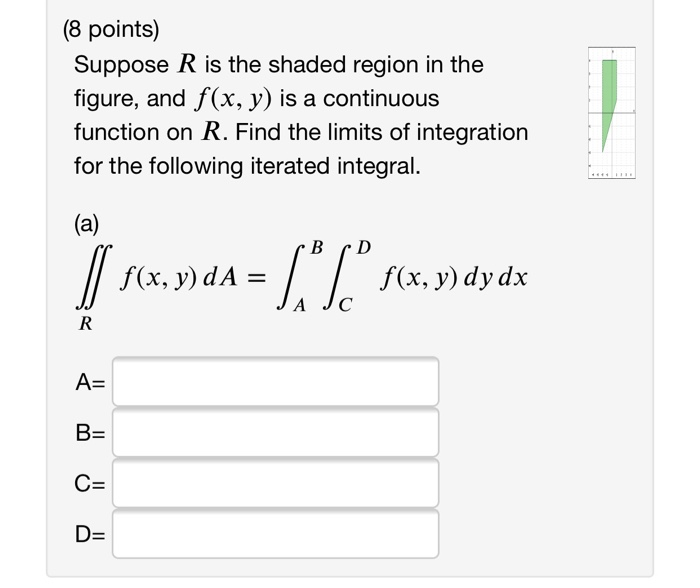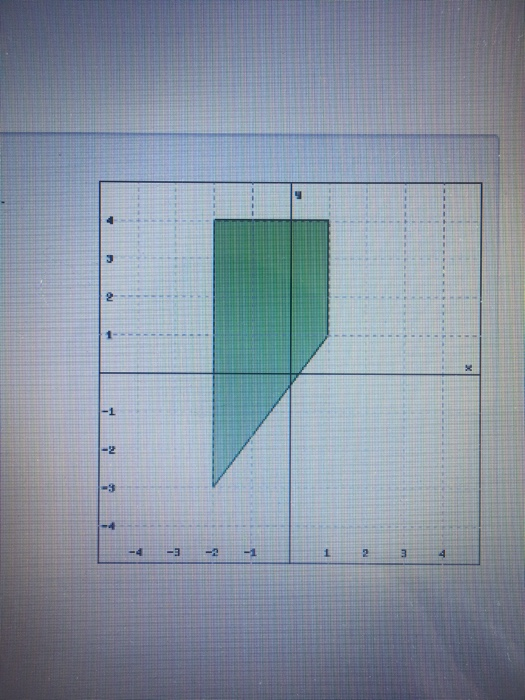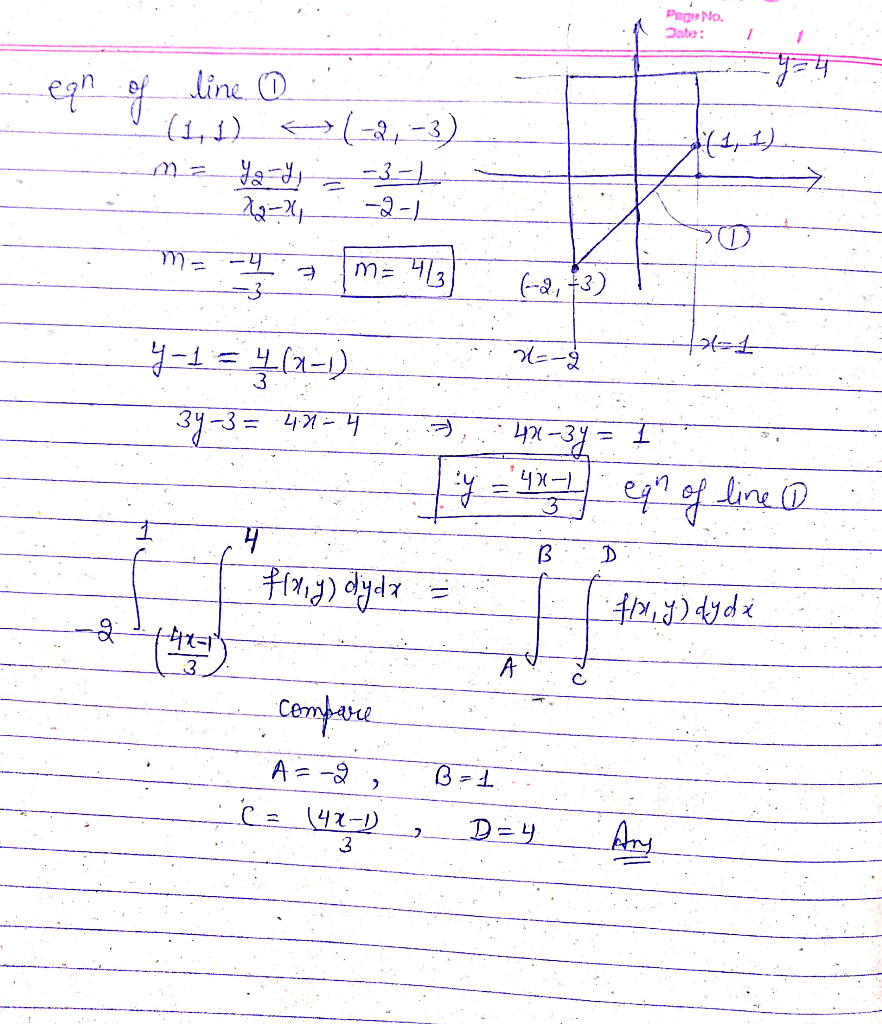Homework Help Question & Answers

(8 points) Suppose R is the shaded region in the figure, and f(x, y) is a continuous function on R. Find the limits of integration for the following iterated integral. fx, y) dy dx JA Jc A-...(8 points) Suppose R is the shaded region in the figure, and f(x, y) is a continuous function on R. Find the limits of integration for the following iterated integral. fx, y) dy dx JA Jc A- D-
3 2 -1 -2 -3 -4 4 -4 3Add Answer of: (8 points) Suppose R is the shaded region in the figure, and f(x, y) is a continuous function on R. Find the limits of integration for the following iterated integral. fx, y) dy dx JA Jc A-...
More Homework Help Questions Additional questions in this topic.

• 5) Given the function fix.y) - x2 and region R bounded by x 0, y x and 2x+y 6 (a) Sketch the region R (label lines, intercepts, axes and shade region) (b) SET UP the integral over this region (c)...

Need Online Homework Help?

Get FREE EXPERT Answers
WITHIN MINUTES
Related Questions